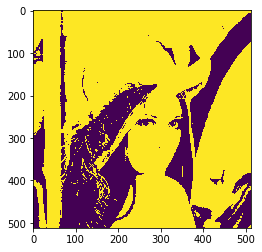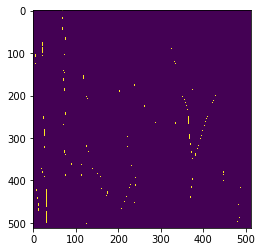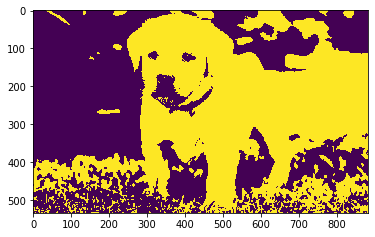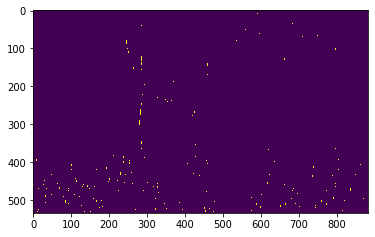# Mahotas – Match template to image exactly

In this article we will see how we can match a template with the image in mahotas. By matching templates we can find the specific location or things in a image with the help of structure we want to find.

In this tutorial we will use “lena” image, below is the command to load it.

`mahotas.demos.load('lena')`

Below is the lena imageIn order to do this we will use mahotas.find method

Syntax : mahotas.find(img, template)

Argument : It takes image object and template as argument

Return : It returns boolean ndarray

Note : Input image should be filtered or should be loaded as grey

In order to filter the image we will take the image object which is numpy.ndarray and filter it with the help of indexing, below is the command to do this

`image = image[:, :, 0]`

Below is the implementation

## Python3

 `# importing required libraries` `import` `mahotas` `import` `mahotas.demos` `from` `pylab ``import` `gray, imshow, show` `import` `numpy as np` `  `  `# loading image` `img ``=` `mahotas.demos.load(``'lena'``)`     `  `  `# filtering image` `img ``=` `img.``max``(``2``)`   `# otsu method` `T_otsu ``=` `mahotas.otsu(img)   ` `  `  `# image values should be greater than otsu value` `img ``=` `img > T_otsu` `  `  `print``(``"Image threshold using Otsu Method"``)`   `# showing image` `imshow(img)` `show()`   `# template` `template ``=` `np.array([` `        ``[``0``, ``1``, ``1``],` `        ``[``0``, ``1``, ``1``],` `        ``[``0``, ``1``, ``1``]], ``bool``)`     `# finding template in the image` `new_img ``=` `mahotas.find(img, template)`   `print``(``"Image with only templates"``)` `# showing new image` `imshow(new_img)` `show()`

Output :

`Image threshold using Otsu Method``Image with only templates`Another example

## Python3

 `# importing required libraries` `import` `mahotas` `import` `numpy as np` `from` `pylab ``import` `gray, imshow, show` `import` `os` ` `  `# loading image` `img ``=` `mahotas.imread(``'dog_image.png'``)`     `# filtering image` `img ``=` `img[:, :, ``0``]` `  `  `# otsu method` `T_otsu ``=` `mahotas.otsu(img)   ` `  `  `# image values should be greater than otsu value` `img ``=` `img > T_otsu` `  `  `print``(``"Image threshold using Otsu Method"``)` `  `  `# showing image` `imshow(img)` `show()`   `# template` `template ``=` `np.array([` `        ``[``0``, ``1``, ``1``],` `        ``[``0``, ``1``, ``1``],` `        ``[``0``, ``1``, ``1``]], ``bool``)`     `# finding template in the image` `new_img ``=` `mahotas.find(img, template)`   `print``(``"Image with only templates"``)` `# showing new image` `imshow(new_img)` `show()`

Output :

`Image threshold using Otsu Method``Image with only templates`Whether you're preparing for your first job interview or aiming to upskill in this ever-evolving tech landscape, GeeksforGeeks Courses are your key to success. We provide top-quality content at affordable prices, all geared towards accelerating your growth in a time-bound manner. Join the millions we've already empowered, and we're here to do the same for you. Don't miss out - check it out now!